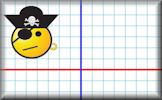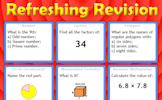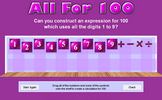# Using graphs

### Term 1 starting in week 5 :: Estimated time: 2 weeks

• Reflect shapes in given lines (review)
• Construct and interpret conversion graphs (review)
• Construct and interpret other real-life straight line graphs (review)
• Interpret distance/time graphs
• Construct distance/time graphs
• Construct and interpret speed/time graphs
• Construct and interpret piece-wise graphs
• Recognise and interpret graphs that illustrate direct and inverse proportion
• Find approximate solutions to equations using graphs

For higher-attaining pupils:

• Estimate the area under a curve

This page should remember your ticks from one visit to the next for a period of time. It does this by using Local Storage so the information is saved only on the computer you are working on right now.

## Lesson Starters

Here are some suggestions for whole-class, projectable resources which can be used at the beginnings of each lesson in this block.

### 1st Lesson#### Reflective Cat

On squared paper copy the drawing of the face then reflect it in three different lines.

### 2nd Lesson#### Book Worm

Work out the distance the book worm needs to eat through to get to the back cover of the last book.

### 3rd Lesson#### Half Hearted

Find the number which when added to the top (numerator) and bottom (denominator) of each fraction make it equivalent to one half.

### 4th Lesson#### Kim's Game 1

This is the Maths version of the traditional memory game. Memorise 10 mathematical facts then recall them as they disappear from view.

### 5th Lesson#### Refreshing Revision

It is called Refreshing Revision because every time you refresh the page you get different revision questions.

### 6th Lesson#### All for 100

Can you write an expression for 100 which uses all the digits 1 to 9?

Some of the Starters above are to reinforce concepts learnt, others are to introduce new ideas while others are on unrelated topics designed for retrieval practice or and opportunity to develop problem-solving skills.

White Rose ResourcesEnd of block assessments provide a quick progress check at the end of each block of learning to make sure students have understood the content covered. This Scheme of Learning was produced by White Rose Maths and is used here with permission granted on 30th June 2021.For All: